Next: LCR Circuits Up: Damped and Driven Harmonic Previous: Damped Harmonic Oscillation

# Quality Factor

The energy loss rate of a weakly damped (i.e.,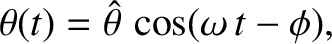) harmonic oscillator is conveniently characterized in terms of a parameter,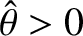, which is known as the quality factor. This quantity is defined to be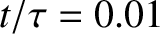times the energy stored in the oscillator, divided by the energy lost in a single oscillation period. If the oscillator is weakly damped then the energy lost per period is relatively small, andis therefore much larger than unity. Roughly speaking,is the number of oscillations that the oscillator typically completes, after being set in motion, before its amplitude decays to a negligible value. For instance, the quality factor for the damped oscillation shown in Figure 7 is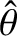. Let us find an expression for.

As we have seen, the motion of a weakly damped harmonic oscillator is specified by [see Equation (72)]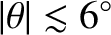(81)

It follows that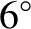(82)

Thus, making use of Equation (78), the energy lost during a single oscillation period is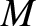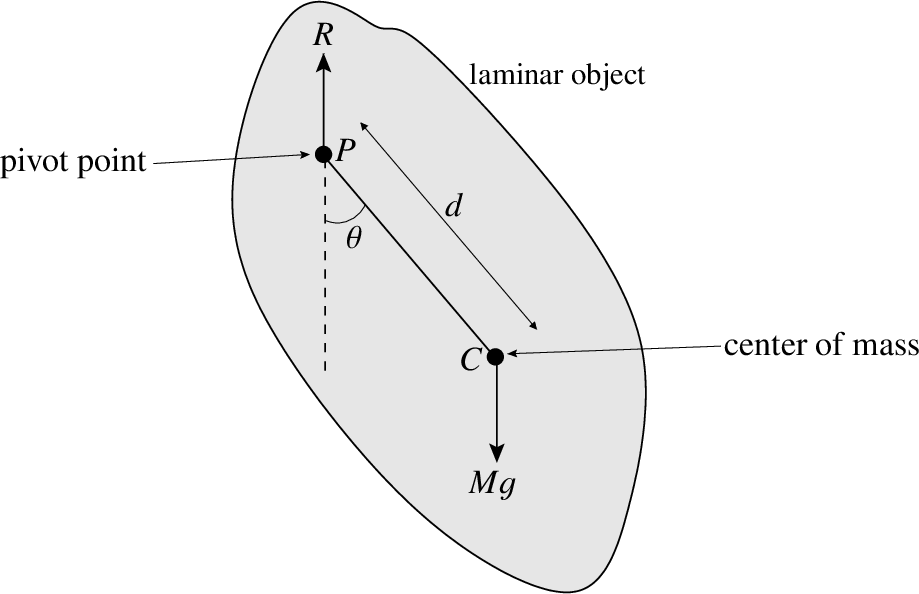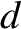(83)

In the weakly damped limit,, the exponential factor is approximately unity in the interval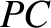to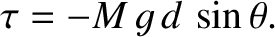, so that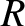(84)

where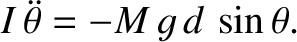. Thus,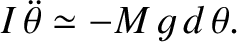(85)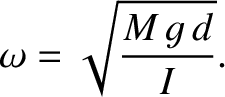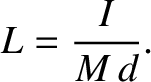(86)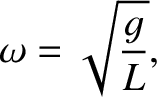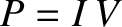(87)

The energy stored in the oscillator (at) is [cf., Equation (16)]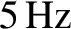(88)

Hence, we obtain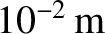(89)Next: LCR Circuits Up: Damped and Driven Harmonic Previous: Damped Harmonic Oscillation
Richard Fitzpatrick 2013-04-08